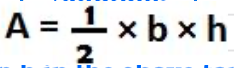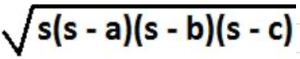Area of a triangle formula will be discussed below:Consider the above triangle formed by joining three points A, B and C. (by the way, three points that do not lie on one same straight line only can form a triangle. And three or more points which lie on a same straight line are called collinear points). Let us call the above figure as triangle ABC and denote it as ∆ABC. The triangle ABC has three sides AB, BC and AC. Let us take the side BC as the base. Let the length of this base be “b”. With respect to side BC as the base, AD will act as height of the triangle ABC. Let its length be “h”. (by the way, what is height? it is the length of the line drawn from vertex A perpendicular to the base BC. The perpendicular line AD touches the base BC at point D) Now, the formula for the area of a triangle ABC denoted as “A” with height “h” and base “b” isNow, let us solve some problems on area of a triangle formula:

Example 1:

The area of a triangular card is 20 sq.cms. Its height is 10 cms. What is the base for this height?

Solution:Let us apply 20 in A and 10 in h in the above formula for Area of a triangle:

20 = ½ × 10 × h, so, h = 20 × 2/10 = 4.

Area of triangle formula for equilateral triangle:

A triangle in which the lengths of all three sides are equal is called an equilateral triangle. Let the length of each side be “a”. Then, the formula for area of an equilateral triangle is:Heron’s formula for area of a triangle. Consider a triangle in which the lengths of the sides are a, b and c. Let s denote the semi-perimeter of the triangle. (peri meter is the sum of the lengths of the three sides in a triangle). Then, the area of a triangle in terms of s, a, b and c is